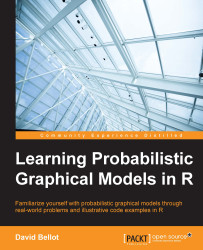•#### Learning Probabilistic Graphical Models in R#### Overview of this book

Probabilistic graphical models (PGM, also known as graphical models) are a marriage between probability theory and graph theory. Generally, PGMs use a graph-based representation. Two branches of graphical representations of distributions are commonly used, namely Bayesian networks and Markov networks. R has many packages to implement graphical models. We’ll start by showing you how to transform a classical statistical model into a modern PGM and then look at how to do exact inference in graphical models. Proceeding, we’ll introduce you to many modern R packages that will help you to perform inference on the models. We will then run a Bayesian linear regression and you’ll see the advantage of going probabilistic when you want to do prediction. Next, you’ll master using R packages and implementing its techniques. Finally, you’ll be presented with machine learning applications that have a direct impact in many fields. Here, we’ll cover clustering and the discovery of hidden information in big data, as well as two important methods, PCA and ICA, to reduce the size of big problems.
Learning Probabilistic Graphical Models in RCreditswww.PacktPub.comPrefaceFree Chapter
Probabilistic ReasoningExact InferenceLearning ParametersBayesian Modeling – Basic ModelsApproximate InferenceBayesian Modeling – Linear ModelsProbabilistic Mixture ModelsAppendixIndex## Chapter 3. Learning Parameters

Building a probabilistic graphical model requires in general three steps: defining the random variables, which are the nodes of the graph as well; defining the structure of the graph; and finally defining the numerical parameters of each local distribution. So far, the last step has been done manually and we have given numerical values to each local probability distribution by hand. In many cases, we have access to a wealth of data and we can find the numerical values of those parameters with a method called parameter learning. In other fields, it is also called parameter fitting or model calibration.

Parameter learning is one important topic in machine learning. In this chapter we will see how we can use a dataset and learn the parameters for a given graphical model. We will go from the simple but common use case, in which the data is fully observable, to a more complex case, in which the data is partially observed, and therefore needs more advanced techniques...